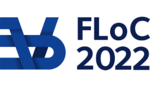FLOC 2022: FEDERATED LOGIC CONFERENCE 2022
TALK KEYWORD INDEX

0
0-1 laws
A
Abstract machines
active learning
Algebraic Circuits
Algebraic Geometry
alphabetic equivalence
Angluin algorithm
arithmetic
Asynchronicity
Automata
automata theory
automatic structures
B
Bar Recursion
Behavioural equivalence
bidirected
bisimilarity
Boolean algebra
bounded expansion
bounded-depth Frege
boundedness problem
C
canonization
categorical semantics
Category
category theory
characterization
chi-boundedness
Choiceless Polynomial Time
circuit complexity
circuit satisfiability
Circular proofs
classical realizability
clique-width
coalgebra
coherence
Coinduction
complete proof system
complexity
complexity analysis
Computable analysis
computational complexity
computer-checked proofs
computing termination probability
Concrete sheaves
Context-free languages
convex polyhedra
Cost models
Cubical Type Theory
Curry-Howard
Curry-Howard correspondence
Cut Elimination
Cyclic proofs
D
data words
dataflow programming
decidability
deciding almost sure termination
Denotational semantics
density
Descriptive Complexity
deterministic hierarchies
dichotomy theorem
distributed automata
Domains
dot-depth hierarchy
E
Eckmann-Hilton
element-free probabilities
equational logic
evaluation
Ewens distribution
expectation transformer
Expected runtime
explicit substitutions
Exponential local-global principle
F
feedback
finite abstraction
Finite Model Theory
first-order logic
first-order theory of the reals
fixed points
Fixed-Point Logic
formal semantics
free algebras
Function algebras
Functor of points
G
Gaifman Normal Form
games
games on graphs
generalised soundness
gluing
graphical calculus
Guarded Recursion
H
higher categories
higher category theory
Higher Inductive Types
Higher order computation
higher-order
homotopy
homotopy type theory
hyperdoctrine
HyperLTL
Hyperproperties
I
Implicit Complexity
Independent Hashing
Induction and coinduction
infinite duration
infinite-domain constraint satisfaction problem
infinite-state systems
Interpretation
interpretations
isolation problem
isomorphism
L
Lafont category
lambda calculus
lambda-calculus
lambda-mu-calculus
linear equations
Linear Integer Arithmetic
linear logic
linear loops
linear real
Linear recurrence sequences
Linear-time/branching-time spectrum
Liveness
local consistency
local-time semantics
Locality
locally presentable category
Logarithmic Space
Logic
logic for PTime
logical relations
Lossy channel systems
lower bounds
LREC
M
Markov categories
membership
metrics
modal mu-calculus
modal type theory
modalities
model checking
model counting
modular circuits
monoidal category
monoidal stream
Mu-Calculus
multinomial theorem
multiset
mutation
N
negotiations
Nondeterminism
normalization
nowhere dense
Nullstellensatz
Number Fields
O
orbit-finite sets
P
PAC learning
parser
partial Horn theory
partial-order methods
partition
path induction
pathwidth
pebble-relation
petri nets
Physical observable
Pigeonhole principle
polymorphism
polynomial closure
Polynomial Identity Testing
positionality
positivity
Presburger
Presburger arithmetic
Preservation theorem
Presheaf Models of Type Theory
probabilistic (affine additive) higher-order recursion schemes
probabilistic programming
probability
probability distribution
probability distributions
process algebra
process graphs
productivity
proof nets
proof-as-program
pushdown
Q
quantifier alternation
quantitative algebra
quantitative reasoning
quantitative/qualitative verification of omega-regular properties
quantum programming
Quantum random walk
Quantum weakest precondition
R
Ramsey quantifier
random interpretations
Randomised Algorithms
randomized computation
reachability
reachability problem
Realizability
recurrent reachability
Recursion
Regular expressions
regular languages
resource approximation
restricted probabilistic tree stack automata
reversible
S
Safe recursion
safety
Satisfiability
Second-Order Arithmetic
semantics
semilinear sets
semiring semantics
semistrict
separation
Sequences of probability distributions
sets with atoms
shrubdepth
Sigma-monoid
signal flow graph
Skolem Conjecture
Skolem Problem
soundness
Space complexity
Stochastic games
stochastic process
Straubing conjecture
stream
strict units
String constraints
structural soundness
Subword order
Sweedler dual
syllepsis
symmetric choice
Synchronization
Synthetic domain theory
synthetic probability
T
Taylor expansion
Team Semantics
Temporal Logic
Termination
thunk-force categories
timed automata
transducers
transduction
transductions
twin-width
two-variable first-order logic
type systems
type theory
U
Undecidability
Uniform Sampling
universal graphs
V
VC dimension